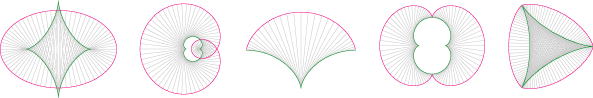# Mathematica Notebooks• GraysProgs.nb

This notebook loads up all the miniprograms written by Alfred Gray to accompany his book on Curves and Surfaces.

• PlanarCurves.nb

Dozens of parametrizations for various curves; programs for computing curvature, length, and winding number; plotting programs for coloring a curve according to its curvature, and programs for plotting curves determined by a given curvature function; several animation programs including cycloid, tractrix, trochoids, involutes, evolutes, the Reuleaux triangle, and wave fronts.

• SpaceCurves.nb

Dozens of parametrizations for various curves; programs for computing curvature, torsion and length; programs for coloring a curve by its curvature or torsion; programs for plotting the tangential, normal, and binormal, spherical images.

• Surfaces.nb

Dozens of parametrizations for various surfaces; programs for computing Gauss and mean curvature; programs for coloring a surface by its Gauss or mean curvature.

• Midpoints.nb

Programs for graphing a sequence of polygons which are generated iteratively by connecting the midpoints of the sides of a given polygon, until a convex figure is produced.

• CrossProducts.nb

How to prove various identities involving dot product and cross products, including the three dimensional version of the Pythagorean theorem.

• ConicSections.nb

Animations of the cross sections of a double cone as planes pass through it in various directions or rotate.

• Bicycle.nb

Animation of a line segment as its midpoint slides around a deltoid and its endpoints trace a convex curve.

• UnfoldingExperiments.nb

An implementation of packages written by Fukuda to unfold a given convex polytope into a nonoverlapping planar regions. In particular, the notebook unfolds all the platonic, archimedean, and Johnson solids. Included also are programs for unfolding convex polytopes generated by random linear inequalites or convex hull of random points.

• NegativeCurvature.nb

This notebook contains all the computations and some of the figures for the paper Topology of negatively curved real affine algebraic surfaces.

• Pseudo-Edge.nb

This notebook contains some computations and the main figure for the paper Pseudo-edge unfoldings of convex polyhedra.

• Spherical-Inspection.nb

This notebook contains the longer computations and some of the figures for the paper Shortest closed curve to inspect a sphere.